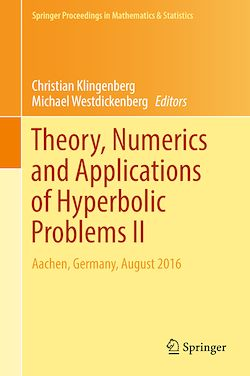# Theory, Numerics and Applications of Hyperbolic Problems II

-English
714 Pages

Description

The second of two volumes, this edited proceedings book features research presented at the XVI International Conference on Hyperbolic Problems held in Aachen, Germany in summer 2016. It focuses on the theoretical, applied, and computational aspects of hyperbolic partial differential equations (systems of hyperbolic conservation laws, wave equations, etc.) and of related mathematical models (PDEs of mixed type, kinetic equations, nonlocal or/and discrete models) found in the field of applied sciences.

Subjects

##### Differential equation

Informations